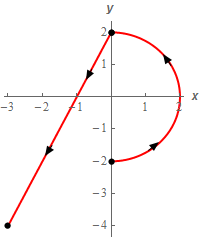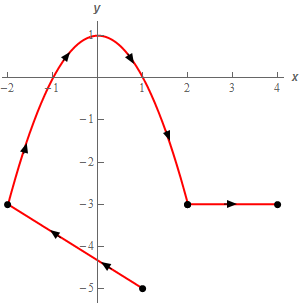Paul's Online Notes
Home / Calculus III / Line Integrals / Line Integrals - Part II
Show Mobile Notice Show All Notes Hide All Notes
Mobile Notice
You appear to be on a device with a "narrow" screen width (i.e. you are probably on a mobile phone). Due to the nature of the mathematics on this site it is best views in landscape mode. If your device is not in landscape mode many of the equations will run off the side of your device (should be able to scroll to see them) and some of the menu items will be cut off due to the narrow screen width.

### Section 16.3 : Line Integrals - Part II

For problems 1 – 5 evaluate the given line integral. Follow the direction of $$C$$ as given in the problem statement.

1. Evaluate $$\displaystyle \int\limits_{C}{{\sqrt {1 + y} \,dy}}$$ where $$C$$ is the portion of $$y = {{\bf{e}}^{2x}}$$ from $$x = 0$$ to $$x = 2$$. Solution
2. Evaluate $$\displaystyle \int\limits_{C}{{2y\,dx + \left( {1 - x} \right)\,dy}}$$ where $$C$$ is portion of $$y = 1 - {x^3}$$ from $$x = - 1$$ to $$x = 2$$. Solution
3. Evaluate $$\displaystyle \int\limits_{C}{{{x^2}\,dy - yz\,dz}}$$ where $$C$$ is the line segment from $$\left( {4, - 1,2} \right)$$ to $$\left( {1,7, - 1} \right)$$. Solution
4. Evaluate $$\displaystyle \int\limits_{C}{{1 + {x^3}\,dx}}$$ where $$C$$ is the right half of the circle of radius 2 with counter clockwise rotation followed by the line segment from $$\left( {0,2} \right)$$ to $$\left( { - 3, - 4} \right)$$. See the sketch below for the direction.Solution
5. Evaluate $$\displaystyle \int\limits_{C}{{2{x^2}\,dy - xy\,dx}}$$ where $$C$$ is the line segment from $$\left( {1, - 5} \right)$$ to $$\left( { - 2, - 3} \right)$$ followed by the portion of $$y = 1 - {x^2}$$ from $$x = - 2$$ to $$x = 2$$ which in turn is followed by the line segment from $$\left( {2, - 3} \right)$$ to $$\left( {4, - 3} \right)$$. See the sketch below for the direction.Solution
6. Evaluate $$\displaystyle \int\limits_{C}{{\left( {x - y} \right)\,dx - y{x^2}\,dy}}$$ for each of the following curves.
1. $$C$$ is the portion of the circle of radius 6 in the 1st, 2nd and 3rd quadrant with clockwise rotation.
2. $$C$$ is the line segment from $$\left( {0, - 6} \right)$$ to $$\left( {6,0} \right)$$.
Solution
7. Evaluate $$\displaystyle \int\limits_{C}{{{x^3}\,dy - \left( {y + 1} \right)\,dx}}$$ for each of the following curves.
1. $$C$$ is the line segment from $$\left( {1,7} \right)$$ to $$\left( { - 2,4} \right)$$.
2. $$C$$ is the line segment from $$\left( { - 2,4} \right)$$ to $$\left( {1,7} \right)$$.
Solution